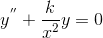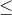# Showing the solution of an Euler DE oscillates

## Homework Statement

I have the DEwhich is an Euler DE. Show that if k >1/4, the solution of the DE would oscillate.

## Homework Equations

eix= cos(x) +isin(x) I assume.

## The Attempt at a Solution

I understand here that if k>1/4 the solution of the DE may oscillate, but if k1/4, it will not. I understand why it would oscillate because the roots of the indicial equation would come on out as complex because if you were to plug the values into the quadratic formula, you would recieve a negative under the square root. Other than explaining it in this fashion, how would some one show this though?

Here I have r=[-1 +/- sqrt(1-4k)]/2